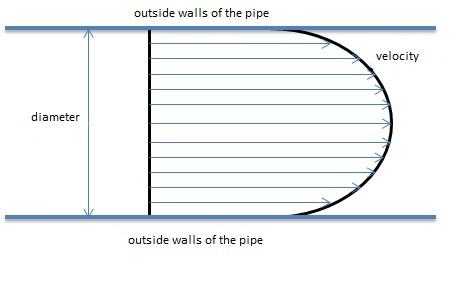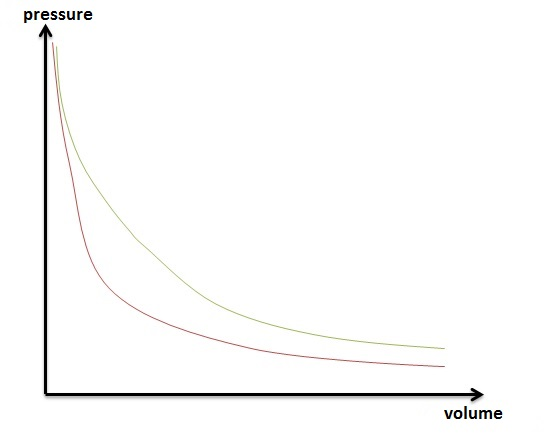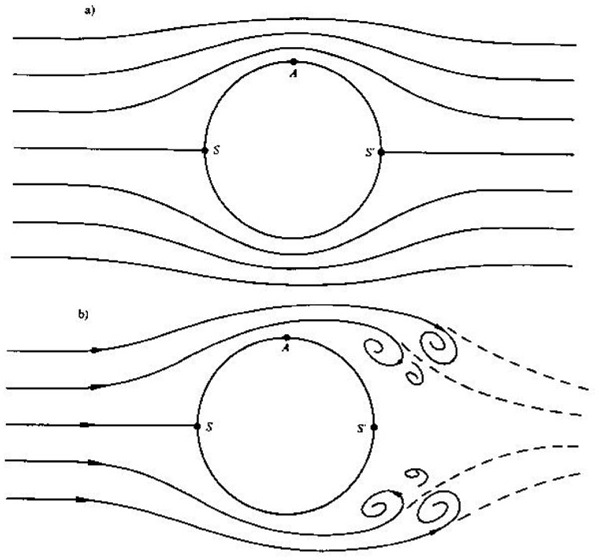Are you more of a visual learner? Check out our online video lectures and start your physics course now for free!Quantity

Volume

Volume is used to describe the contents of a 3-dimensional space. Volume is expressed in liters (L) in addition to the SI unit m³. The calculation of volume varies depending on the type of body.

In case of a cylinder, the base of a cylinder is a circle, the area of which is calculated as:

A = π * r2

Based on the formula for the volume (V = A * h) the following equation is used for a cylinder:

Vcylinder = A * h = π * r2 * h

V ⇒ Volume [m³]

Mass

Mass is a property of matter:

• Inertial mass provides resistance to the body during accelerated movement;
• Gravitational mass is a measure of how heavy a body is.

Mass of a body can be measured with a scale. In some cases, the mass can be calculated based on the principle of conservation of momentum. However, the calculation of mass varies from case to case.

m ⇒ mass [kg]

Particle count

The particle count (or the number of particles) corresponds to the absolute sum of particles within a system. It is directly proportional to the amount of substance. In macroscopically small systems, in which the particle count cannot be correctly determined, the amount of substance can be calculated using the Avogadro’s constant:

N = n * NA

N ⇒ number of particles, no unit
n ⇒ amount of substance [mole]

N.B. One mole of a substance always contains NA= 6,02 * 1023 particles of this substance.

Amount of substance and molar mass

The amount of substance provides indirect information regarding the particle count of a system. It is stated in the unit ‘mole.’ The molar mass is required to establish the masses of a substance during an experiment. The atomic mass in a reaction can be found in the periodic table of the elements.

The amounts required during a reaction can be calculated with the following formula:

m = n * M

n ⇒ amount of substance [mole]
M ⇒ molar mass [g/mole]

Example: Magnesium oxide is generated by the reaction between magnesium and oxygen (the ‘burning of magnesium’).

2 Mg + O2 → 2 MgO

According to the reaction equation, two moles of magnesium and one mole of oxygen are required to form 2 moles of magnesium oxide. The molar masses of the substances involved are:

Magnesium: 24.31 g/mol; oxygen: 15.9994 g/mol.

Incorporating the values of molar mass in the above equation, m = n * M, the following results are obtained:

Magnesium: m = 2 mole * 24.31 g/mol = 48.62 g

Oxygen: m = 1 mole * 15.9994 g/mol = 15.9994 g

Thus, for this reaction, you require 48.62 g of magnesium and 15.9994 g of oxygen.

Densities

Under constant external conditions, the mass of a body is directly proportional to the volume and is derived from the quotient of mass and volume. At certain temperatures and at constant pressure, the quotient of mass and volume is characteristic of a specific substance. It is called density:

ρ = m / V

ρ ⇒ density (mass density) [kg/cm³]

The density of solid, liquid, and gaseous substances depends on the temperature. The density of gaseous bodies also depends on the pressure.

Particle density

The particle density is defined as the quotient of particle count and volume. The particle count indicates the density of the substance:

Ci = Ni/V

Ci ⇒ particle density [particles/cm³]
Ni ⇒ particle count of a specific substance

Mass-based sizes

Specific volume

The specific volume is defined as the reciprocal of the density and depends on the volume of the unit of mass as shown in the following equation:

V = 1/p = V/m

v ⇒ specific volume [m³/kg]

Among other things, it is used to create P-V diagrams in thermodynamics, which describe changes in volume and pressure in a system. A P-V diagram is presented below.Image by Lecturio

Specific heat capacity

The increase in the temperature of a body causes an increase in the kinetic energy of its smallest particles. Heating entails energy input, and cooling involves energy extraction. The heat absorbed by a body is proportional to the mass and temperature change of the body. The constant of proportionality is called specific heating capacity. It is a material constant.

Example: The specific heating capacity for water is cH2O = 4.19 J/(kg*K), i.e., the energy of 4.19 kJ is necessary to increase 1 kg of water by 1 K.

The specific heating capacity, or the heat, is calculated as:

ΔQ = c * m * ΔT

c ⇒ specific heating capacity [J/(kg*K]
ΔQ ⇒ heat output/intake
ΔT ⇒ increase in temperature/decrease in temperature

Substance mixtures

In nature (including within the human body), the matter is rarely encountered as a pure substance. Substances bind together and depending on the bond, they exhibit different features. Specific combinations of substances, such as cholesterol or calcium phosphate deposits, increase the resistance to blood flow, resulting in arteriosclerosis.

However, substance mixtures may also be useful, for instance, when the iron is formed, stored and released as needed in the liver in the form of ferritin crystals.

Mole fraction

The mole fraction is the amount of substance based on the number of moles in a gas or liquid mixture.

Example: A mole of air is based on 80% nitrogen and 20% oxygen. Therefore, the mole fractions are 0.2 oxygen and 0.8 nitrogen.

Mass fraction

A mass fraction is the proportion of a dissolved substance relative to the entire mass of the solution.

States of matter

The states of matter define the physical states of a substance depending on temperature and pressure. The 3 different states include:

• Solid, containing a fixed alignment and bond between the atoms.
• Liquid, comprising mobile and unorganized atoms.
• Gas, with almost no bond between the atoms.

Flow of liquids and gases

Blood pressure is a measure of the dynamic pressure in the blood. If this pressure is too high, it can be reduced by increasing the speed of flow, i.e., either by increasing the volume flow or lowering the flow resistance.

The expiratory air must pass through a narrowed portion or glottis in the vocal cords. Rheology is the study of deformation and non-Newtonian flow of liquids as well as the plastic flow of solids. It can be used to describe how high the flow resistance or the volume flow must be to open or close the glottis, the relationships between those factors, and the Bernoulli equation states.

Flow fields

The flow of ideal liquids and gases is illustrated with streamlines. The streamlines are thicker at higher flow speeds.

1. Laminar flow: In a laminar flow, despite obstructions or constrictions, the lines do not cease but rather continue onward.
2. Turbulent flow: The turbulent flow is characterized by cessation of flow due to obstructions or constrictions.Flow resistance and Hagen-Poiseuille’s law

If a body is placed in a liquid, it is subjected to a force that, in many cases, turns out to be proportional to the density of the fluid, to the square of the flow speed, and to the cross-sectional area.

Newton’s Law states that every force is counteracted by an equal force (Newton’s action-reaction principle). This counteracting force is described as the resistance of a body and is dependent on viscosity, internal friction, and obstructions in the flow.

The flow resistance is calculated using the Hagen-Poiseuille equation:

RS = 8π * η * Δl / A2

η ⇒ viscosity of the fluid [(n*s) / m²]
Δl ⇒ length of the flow / length of the pipe [m]
A ⇒ cross-section [m²]

Volume flow rate

The volume flow rate states how much volume per unit of time flows through a cross-section. It is defined by the following equation:

V = A / Δt * Δl

V ⇒ volume flow rate [m³/s]

Continuity equation

During laminar flow, the number of streamlines remains constant. If the cross-section of the pipe is smaller, the streamlines are condensed, i.e., the flow speed is increased.

The smaller the cross-sectional area, the greater the flow speed as the volume flow rate remains constant.

V1 = V2

The flow speeds are thus inversely correlated with the pipe cross-section.

Bernoulli’s equation

When a liquid with a specific density flows horizontally through a pipe with changing cross-section–and provided that friction is insignificant–the total pressure remains constant in all parts of the pipe:

p1 = 0,5ρ * v12 = p2 + 0,5ρ * v 22

In slanted pipes, the sum of static pressure, hydrostatic pressure, and dynamic pressure is constant at every point on a streamline.

Ohm’s law and real fluids

In contrast to ideal fluids, real fluids undergo a loss in pressure through internal friction or viscosity. Friction always induces a loss in kinetic energy, resulting in the fluid adhering to the walls of the pipe.

Therefore, a fluid also flows more slowly at the edges. In comparison, the flow speed in the middle of the flow is greater. The graphic course of such a fluid resembles a parabola:The zenith of the parabolic course of the flow speed is in the center. Fluids that exhibit such a graphic course during flow in cylindrical pipes are called Newtonian fluids. Ohm’s law applies to such fluids:

V = Δρ / RS

A linear relationship exists between the volume flow rate and the pressure difference.

Series circuit of flow resistance

As described above, the pressure in real fluids decreases and thus sinks with the length of the pipe; it is also dependent on the cross-section of the pipe. The flow resistance is similar to the resistance in an electrical system and is thus cumulative.

Kirchhoff’s laws

Pipes through which fluids flow can be connected together:

• Consecutively, i.e., in a row, as the resistance is added together.
• Branching, in which the total flow is constant at each branch node, i.e., the total incoming liquid is equal to the total outgoing liquid.
Learn. Apply. Retain.
Your path to achieve medical excellence.
Study for medical school and boards with Lecturio.